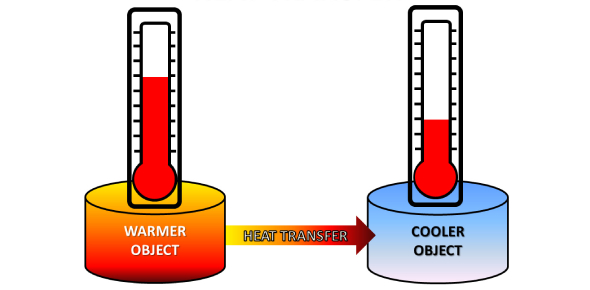# Heat Transfer Trivia Quiz!

19 Questions | Attempts: 252
ShareSettings.

• 1.
Thermal conductivity of water in general with rising in temperature.
• A.

Increases

• B.

Decreases

• C.

Remains constant

• D.

May increase or decrease depending on temperature

• E.

None of the above.

• 2.
Heat transfer takes place as per_____________
• A.

Zeroth law of thermodynamics

• B.

First law of thermodynamic

• C.

Second law of the thermodynamics

• D.

Kirchhoff law

• E.

Stefan's law.

• 3.
Thermal conductivity of air with rising in temperature:
• A.

Increases

• B.

Decreases

• C.

Remains constant

• D.

May increase or decrease depending on temperature

• E.

None of the above.

• 4.
The capacity to do work is called as:
• A.

Heat

• B.

Energy

• C.

work

• D.

None of the above

• 5.
Heat is measured in:
• A.

Joule

• B.

Calorie

• C.

Both A and B

• D.

Joule/second

• 6.
The form of energy that produces a feeling of hotness is called?
• A.

Work

• B.

Heat

• C.

Energy

• D.

none of the above

• 7.
With increase in temperature, heat will be:
• A.

Increase

• B.

Constant

• C.

Dcrease

• D.

Double

• 8.
Heat capacity depends on?
• A.

Change in temperature

• B.

Mass of body

• C.

Nature of substance

• D.

All the above

• 9.
Which of the following are the processes of transfer of heat?
• A.

Conduction

• B.

Convection

• C.

• D.

All the above

• 10.
The process of transfer of heat in solids is called as:
• A.

Conduction

• B.

Convection

• C.

• D.

All the above

• 11.
When heat is transferred from one particle of a hot body to another by actual motion of the heated particles, it is referred to as heat transfer by?
• A.

Conduction

• B.

Convection

• C.

• D.

Conduction and convection

• E.

All the above

• 12.
In Conduction process the molecules of the solid pass the heat from one to another:
• A.

Without themselves moving from one place to another.

• B.

Without themselves moving from their positions.

• C.

Themselves move from one place to another

• D.

None of the above

• 13.
Unit of thermal conductivity in M.K.S. units is:
• A.

Kcal/kg m2 °c

• B.

Kcal-m/hr m2 °C

• C.

Kcal/hr m2 °C

• D.

Kcal-m/hr °C

• 14.
In convection, the molecules:
• A.

Without themselves moving from their positions.

• B.

Themselves move from one place to another

• C.

Without themselves moving from one place to another.

• D.

None of the above

• 15.
Solids are not heated by convection because:
• A.

Solid are not free to move from one place to another

• B.

Molecules only vibrate about fixed position

• C.

Both A and B

• D.

None of the above

• 16.
It is the process of heat transfer from a hot body to a colder body without heating the space between the two is called as:
• A.

Conduction

• B.

Convection

• C.

• D.

Absorption

• 17.
At what factor heat absorbed on radiation by body depends on?
• A.

Distance between body

• B.

Source of heat

• C.

Its colour

• D.

All the above

• 18.
Heat of sun reach the earth by :
• A.

Conduction

• B.

Convection

• C.

• D.

Absorption

• 19.
Clothes of ………………… colours absorb heat better than clothes of ………….colours.
• A.

Light, dark

• B.

Dark, light

• C.

Soft, dark,

• D.

None of the above

## Related TopicsBack to top
×

Wait!
Here's an interesting quiz for you.